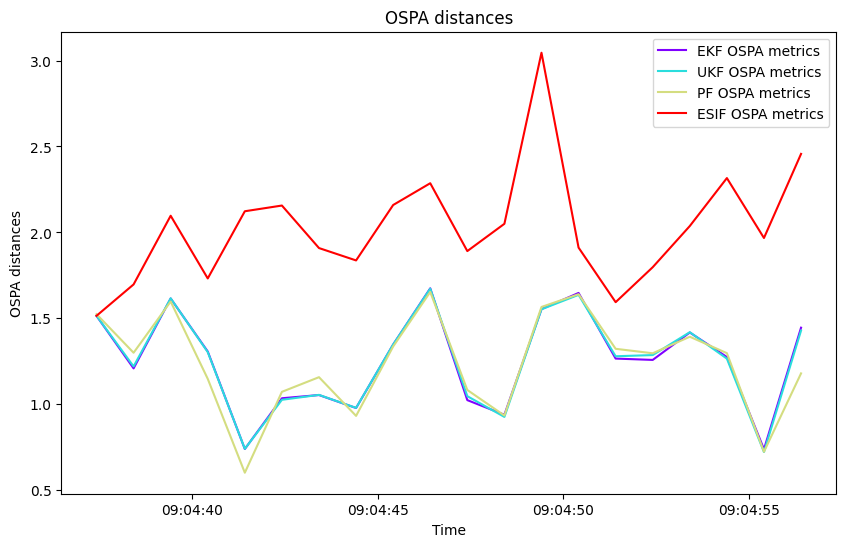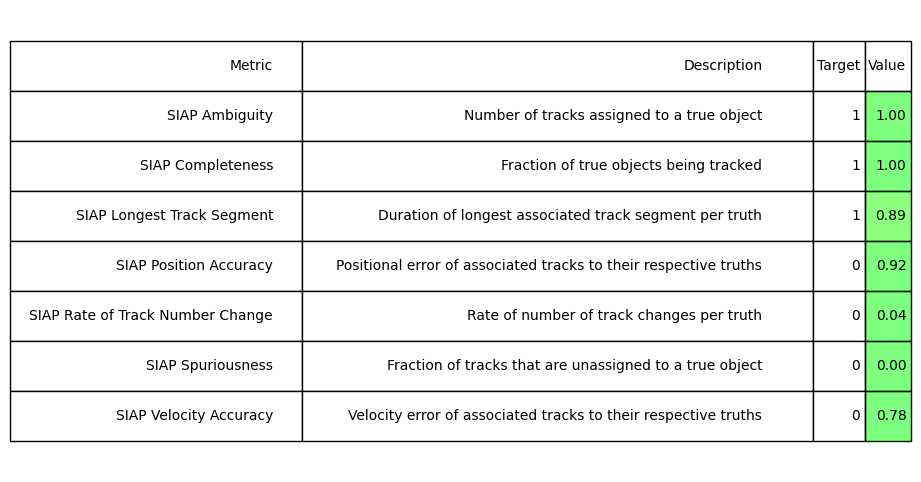# Comparing Multiple Trackers On Manoeuvring Targets

This example shows how multiple trackers can be compared against each other using the same set of detections.

This notebook creates groundtruth with manoeuvring motion, generates detections using a sensor, and attempts to track the groundtruth using the Extended Kalman Filter (EKF), Unscented Kalman Filter (UKF), the Particle Filter (PF), and the Extended Sliding Innovation Filter (ESIF). Each of these trackers assumes a constant velocity transition model. The trackers are compared against each other using distance-error metrics, with the capability of displaying other metrics for the user to explore. The aim of this example is to display the effectiveness of different trackers when tasked with following a manoeuvring target with non-linear detections.

## Layout

The layout of this example is as follows:

1. The ground truth is created using multiple transition models

2. The non-linear detections are generated once per time step using a bearing-range sensor

3. Each tracker is initialised and run on the detections

4. The results are plotted, and tracking metrics displayed for the user to explore

## 1) Create Groundtruth

Firstly, we initialise the ground truth states:

import numpy as np
import datetime
from stonesoup.types.array import StateVector, CovarianceMatrix
from stonesoup.types.state import State, GaussianState

start_time = datetime.datetime.now()
np.random.seed(2)
initial_state_mean = StateVector([, , , ])
initial_state_covariance = CovarianceMatrix(np.diag([4, 0.5, 4, 0.5]))
timestep_size = datetime.timedelta(seconds=5)
number_steps = 20
initial_state = GaussianState(initial_state_mean, initial_state_covariance)


Next, we initialise the transition models used to generate the ground truth. Here, we say that the targets will mostly go straight ahead with a constant velocity, but will sometimes turn left or right. This is implemented using the SwitchMultiTargetGroundTruthSimulator.

from stonesoup.models.transition.linear import (
CombinedLinearGaussianTransitionModel, ConstantVelocity, KnownTurnRate)

# initialise the transition models the ground truth can use
constant_velocity = CombinedLinearGaussianTransitionModel(
[ConstantVelocity(0.05), ConstantVelocity(0.05)])

# create a probability matrix - how likely the ground truth will use each transition model,
# given its current model
model_probs = np.array([[0.7, 0.15, 0.15],  # keep straight, turn left, turn right
[0.4, 0.6, 0.0],  # go straight, keep turning left, turn right
[0.4, 0.0, 0.6]])  # go straight, turn left, keep turning right

from stonesoup.simulator.simple import SwitchMultiTargetGroundTruthSimulator
from stonesoup.types.state import GaussianState
# generate truths

n_truths = 3
xmin = 0
xmax = 40
ymin = 0
ymax = 40
preexisting_states = []

for i in range(0, n_truths):
x = np.random.randint(xmin, xmax) - 1  # x position of initial state
y = np.random.randint(ymin, ymax) - 1  # y position of initial state
y_vel = np.random.randint(-20, 20) / 10  # x velocity will start between -2 and 2
x_vel = np.random.randint(-20, 20) / 10  # y velocity will start between -2 and 2
preexisting_states.append(StateVector([x, x_vel, y, y_vel]))


Now we have initialised everything, so we can generate the ground truth:

ground_truth_gen = SwitchMultiTargetGroundTruthSimulator(
initial_state=initial_state,
transition_models=[constant_velocity, turn_left, turn_right],
model_probs=model_probs,  # put in matrix from above
number_steps=number_steps,  # how long we want each track to be
birth_rate=0,
death_probability=0,
preexisting_states=preexisting_states
)


This has created ground truth that has some twists and turns in it, which we will use to generate detections.

## 2) Generate detections using a bearing-range sensor

The next step is to create a sensor and use it to generate detections from the targets. The sensor we use in this example is a radar with imperfect measurements in bearing-range space.

from stonesoup.sensor.radar import RadarBearingRange

# Create the sensor
ndim_state=4,
position_mapping=[0, 2],  # Detecting x and y
clutter_model=None,  # Can add clutter model in future if desired
)


Now we place the sensor into the simulation:

from stonesoup.platform import FixedPlatform
platform = FixedPlatform(State(StateVector([20, 0, 0, 0])), position_mapping=[0, 2],
sensors=[sensor])


Now we run the sensor and create detections:

from itertools import tee
from stonesoup.simulator.platform import PlatformDetectionSimulator

detector = PlatformDetectionSimulator(ground_truth_gen, platforms=[platform])
detector, *detectors = tee(detector, 6)
# Enables multiple trackers to run on the same detections


We put the detections and ground truths into sets so that we can plot them:

detections = set()
ground_truth = set()

for time, dets in detector:
detections |= dets
ground_truth |= ground_truth_gen.groundtruth_paths


And now we plot the ground truth and detections:

from stonesoup.plotter import Plotterly

plotter = Plotterly()
plotter.plot_ground_truths(ground_truth, [0, 2])
plotter.plot_measurements(detections, [0, 2])
plotter.plot_sensors(sensor)
plotter.fig


## 3) Initialise and run each tracker on the detections

With the detections now generated, our focus turns to creating and running the trackers. This section of the notebook is quite long because each tracker requires an initiator, deleter, detector, data associator, and updater. However, all of these things are standard stonesoup building blocks.

Firstly, we approximate the transition model of the target. Here we assume a constant velocity model, which will be wrong due to the fact that we designed the targets to sometimes turn left or right. We do this to test how effectively each tracking algorithm can perform against target behaviour that doesn’t move exactly as predicted.

transition_model_estimate = CombinedLinearGaussianTransitionModel([ConstantVelocity(0.5),
ConstantVelocity(0.5)])
# Tracking algorithm incorrectly estimates the type of path the truth takes


Next, we initialise the predictors, updaters, hypothesisers, data associators, and deleter. The particle filter requires a resampler as part of its updater. Note that the ESIF is a slight extension of the EKF and uses an EKF predictor.

from stonesoup.predictor.kalman import ExtendedKalmanPredictor, UnscentedKalmanPredictor
from stonesoup.predictor.particle import ParticlePredictor
# introduce the predictors
predictor_EKF = ExtendedKalmanPredictor(transition_model_estimate)
predictor_UKF = UnscentedKalmanPredictor(transition_model_estimate)
predictor_PF = ParticlePredictor(transition_model_estimate)

# ######################################################################

from stonesoup.resampler.particle import ESSResampler
resampler = ESSResampler()

# ######################################################################

from stonesoup.updater.kalman import ExtendedKalmanUpdater, UnscentedKalmanUpdater
from stonesoup.updater.slidinginnovation import ExtendedSlidingInnovationUpdater
from stonesoup.updater.particle import ParticleUpdater
# introduce the updaters

updater_EKF = ExtendedKalmanUpdater(sensor)
updater_UKF = UnscentedKalmanUpdater(sensor)
updater_ESIF = ExtendedSlidingInnovationUpdater(measurement_model=None,
layer_width=10 * np.diag(sensor.noise_covar))
updater_PF = ParticleUpdater(measurement_model=None, resampler=resampler)

# ######################################################################

from stonesoup.hypothesiser.distance import DistanceHypothesiser
from stonesoup.measures import Mahalanobis
# introduce the hypothesisers

hypothesiser_EKF = DistanceHypothesiser(predictor_EKF, updater_EKF,
measure=Mahalanobis(), missed_distance=4)
hypothesiser_UKF = DistanceHypothesiser(predictor_UKF, updater_UKF,
measure=Mahalanobis(), missed_distance=4)
hypothesiser_ESIF = DistanceHypothesiser(predictor_EKF, updater_ESIF,
measure=Mahalanobis(), missed_distance=4)
hypothesiser_PF = DistanceHypothesiser(predictor_PF, updater_PF,
measure=Mahalanobis(), missed_distance=4)

# ######################################################################

from stonesoup.dataassociator.neighbour import GNNWith2DAssignment
# introduce the data associators

data_associator_EKF = GNNWith2DAssignment(hypothesiser_EKF)
data_associator_UKF = GNNWith2DAssignment(hypothesiser_UKF)
data_associator_ESIF = GNNWith2DAssignment(hypothesiser_ESIF)
data_associator_PF = GNNWith2DAssignment(hypothesiser_PF)

# ######################################################################

from stonesoup.deleter.time import UpdateTimeDeleter
# create a deleter
deleter = UpdateTimeDeleter(datetime.timedelta(seconds=5), delete_last_pred=True)


Now we introduce the initial predictors which will be used in the data associator in the track initiators:

init_transition_model = CombinedLinearGaussianTransitionModel(
(ConstantVelocity(1), ConstantVelocity(1)))
init_predictor_EKF = ExtendedKalmanPredictor(init_transition_model)
init_predictor_UKF = UnscentedKalmanPredictor(init_transition_model)
init_predictor_PF = ParticlePredictor(init_transition_model)


The final step before running the trackers is to create the initiators:

from stonesoup.initiator.simple import MultiMeasurementInitiator

initiator_EKF = MultiMeasurementInitiator(
GaussianState(
np.array([, , , ]),  # Prior State
np.diag([1, 1, 1, 1])),
measurement_model=None,
deleter=deleter,
data_associator=GNNWith2DAssignment(
DistanceHypothesiser(init_predictor_EKF, updater_EKF, Mahalanobis(), missed_distance=5)),
updater=updater_EKF,
min_points=2
)

# ######################################################################

initiator_UKF = MultiMeasurementInitiator(
GaussianState(
np.array([, , , ]),  # Prior State
np.diag([1, 1, 1, 1])),
measurement_model=None,
deleter=deleter,
data_associator=GNNWith2DAssignment(
DistanceHypothesiser(init_predictor_UKF, updater_UKF, Mahalanobis(), missed_distance=5)),
updater=updater_UKF,
min_points=2
)

# ######################################################################

initiator_ESIF = MultiMeasurementInitiator(
GaussianState(
np.array([, , , ]),  # Prior State
np.diag([1, 1, 1, 1])),
measurement_model=None,
deleter=deleter,
data_associator=GNNWith2DAssignment(
DistanceHypothesiser(init_predictor_EKF, updater_ESIF, Mahalanobis(), missed_distance=5)),
updater=updater_ESIF,
min_points=2
)


The initiator for the particle filter works differently, so is shown below for clarity:

from stonesoup.initiator.simple import GaussianParticleInitiator
from stonesoup.types.state import GaussianState
from stonesoup.initiator.simple import SimpleMeasurementInitiator

prior_state = GaussianState(
StateVector([20, 0, 10, 0]),
np.diag([1, 1, 1, 1]) ** 2)

initiator_Part = SimpleMeasurementInitiator(prior_state, measurement_model=None,
skip_non_reversible=True)
initiator_PF = GaussianParticleInitiator(number_particles=2000,
initiator=initiator_Part,
use_fixed_covar=False)


Now we run the trackers and store the tracks in sets for plotting:

from stonesoup.tracker.simple import MultiTargetTracker

kalman_tracker_EKF = MultiTargetTracker(  # Runs the tracker
initiator=initiator_EKF,
deleter=deleter,
detector=detectors,
data_associator=data_associator_EKF,
updater=updater_EKF,
)

tracks_EKF = set()
for step, (time, current_tracks) in enumerate(kalman_tracker_EKF, 1):
tracks_EKF.update(current_tracks)

# Stores the tracks in a set for plotting

# #######################################################################

kalman_tracker_UKF = MultiTargetTracker(
initiator=initiator_UKF,
deleter=deleter,
detector=detectors,
data_associator=data_associator_UKF,
updater=updater_UKF,
)

tracks_UKF = set()
for step, (time, current_tracks) in enumerate(kalman_tracker_UKF, 1):
tracks_UKF.update(current_tracks)

# ##########################################################################

kalman_tracker_ESIF = MultiTargetTracker(
initiator=initiator_ESIF,
deleter=deleter,
detector=detectors,
data_associator=data_associator_EKF,
updater=updater_ESIF,
)

tracks_ESIF = set()
for step, (time, current_tracks) in enumerate(kalman_tracker_ESIF, 1):
tracks_ESIF.update(current_tracks)

# ##########################################################################

tracker_PF = MultiTargetTracker(
initiator=initiator_PF,
deleter=deleter,
detector=detectors,
data_associator=data_associator_PF,
updater=updater_PF,
)

tracks_PF = set()
for step, (time, current_tracks) in enumerate(tracker_PF, 1):
tracks_PF.update(current_tracks)


Finally, we plot the results:

plotter.plot_tracks(tracks_EKF, [0, 2], track_label="EKF", line=dict(color="orange"),
uncertainty=False)
plotter.plot_tracks(tracks_UKF, [0, 2], track_label="UKF", line=dict(color="blue"),
uncertainty=False)
plotter.plot_tracks(tracks_PF, [0, 2], track_label="PF", line=dict(color="brown"),
uncertainty=False)
plotter.plot_tracks(tracks_ESIF, [0, 2], track_label="ESIF", line=dict(color="green"),
uncertainty=False)
plotter.fig


## 4) Calculate and display metrics to show effectiveness of different tracking algorithms

The final part of this example is to calculate metrics that can determine how well each tracking algorithm followed the target. None will be perfect due to the sensor measurement noise and error in data association where multiple tracks meet, but some will perform better than others.

This section of the example follows code from the metrics example, which is also used in the sensor management tutorials. More complete documentation can be found there.

Firstly, we calculate the Optimal Sub-Pattern Assignment (OSPA) distance at each time step for each tracker. This is a measure of how far the calculated tracks are from the ground truth. We first initialise the metrics before plotting:

tracking_filters = ['EKF', 'UKF', 'PF', 'ESIF']

from stonesoup.metricgenerator.ospametric import OSPAMetric

ospa_generators = [OSPAMetric(c=40, p=1,
generator_name=f'{tracking_filter} OSPA metrics',
tracks_key=f'tracks_{tracking_filter}',
truths_key='truths'
)
for tracking_filter in tracking_filters]

from stonesoup.metricgenerator.tracktotruthmetrics import SIAPMetrics
from stonesoup.measures import Euclidean

siap_generators = [SIAPMetrics(position_measure=Euclidean((0, 2)),
velocity_measure=Euclidean((1, 3)),
generator_name=f'{tracking_filter} SIAP metrics',
tracks_key=f'tracks_{tracking_filter}',
truths_key='truths'
)
for tracking_filter in tracking_filters]

from stonesoup.metricgenerator.uncertaintymetric import SumofCovarianceNormsMetric

uncertainty_generators = [
SumofCovarianceNormsMetric(generator_name=f'{tracking_filter} OSPA metrics',
tracks_key=f'tracks_{tracking_filter}')
for tracking_filter in tracking_filters]


Now we initialise the metric manager and generate the metrics:

from stonesoup.dataassociator.tracktotrack import TrackToTruth
from stonesoup.metricgenerator.manager import MultiManager

associator = TrackToTruth(association_threshold=30)

generators = ospa_generators + siap_generators + uncertainty_generators
metric_manager = MultiManager(generators, associator=associator)

'tracks_EKF': tracks_EKF,
'tracks_UKF': tracks_UKF,
'tracks_PF': tracks_PF,
'tracks_ESIF': tracks_ESIF
})
metrics = metric_manager.generate_metrics()


Now we can plot the OSPA distance for each tracker:

from stonesoup.plotter import MetricPlotter

fig1 = MetricPlotter()
fig1.plot_metrics(metrics, metric_names=['OSPA distances'])It can be seen that the EKF, UKF, and Particle Filter all behave very similarly, whereas the ESIF has very poor relative performance. A singular performance metric is calculated from this by summing the OSPA value over all timesteps:

# sum up distance error from ground truth over all timestamps
for tracking_filter in tracking_filters:
total = sum([metrics[f'{tracking_filter} OSPA metrics']['OSPA distances'].value[i].value
for i in range(0, len(metrics[f'{tracking_filter} OSPA metrics']['OSPA distances'].value))])
print(f'OSPA total value for {tracking_filter} is {total:.3f}')

OSPA total value for EKF is 25.037
OSPA total value for UKF is 25.040
OSPA total value for PF is 25.674
OSPA total value for ESIF is 40.578


Finally, we calculate the SIAP metrics for the EKF. The same metrics can be calculated for the other trackers if desired. The user can copy this section of code and replace the relevant variable names to get the full metrics for the UKF, ESIF, and Particle Filter.

from stonesoup.metricgenerator.metrictables import SIAPTableGenerator

# generate metrics for EKF
siap_metrics = metrics['EKF SIAP metrics']
siap_averages_EKF = {siap_metrics.get(metric) for metric in siap_metrics
if metric.startswith("SIAP") and not metric.endswith(" at times")}

_ = SIAPTableGenerator(siap_averages_EKF).compute_metric()
print("\n\nSIAP metrics for EKF:")SIAP metrics for EKF:


Total running time of the script: (0 minutes 6.891 seconds)

Gallery generated by Sphinx-Gallery